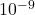## the charge density in an insulateed solid sphere of radius find the electric field at a distance of from the center of the solid

Question

the charge density in an insulateed solid sphere of radius find the electric field at a distance of from the center of the solid

in progress 0
1 month 2021-08-07T20:13:08+00:00 1 Answers 1 views 0

Assuming the charged density in the insulated solid sphere of radius 3.1m is 8.8e-9, the electric field at 5.2 meters is 73.1256.

Explanation:

The electric charge linear density is equal to 8.8 xthe radius of the sphere is 3.1m

The magnitude of the electric field at the radius of the sphere equal to 5.2 meters can be calculated with the formula ;

– E = λ / 4πε₀ [ r  / α ( α + r ) ]Solution:

E =  8.8 x/ 4πε₀ [ 3.1/ 5.2( 5.2 + 3.1) ]= 1018.0995 [0.07183]=  73.1256